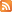Entries (RSS) -Comments (RSS)

### Hello 9-05

Since you seem to be the class and the only ones communicating in this way I thought I should let you know that I have posted a site to help you understand the difference between Similar and Congruent triangles. Check our class definitions today to make sure we have them right and then also check the extra definitions.

I need to go over the problems of the week for chapter 3 with you. Please remind me to do that. Here are some more problems for you to look at for chapter 4. For question 2 borrow some blocks from me to help you understand the relationship.

Chapter 4 Problems

1. A camera negative is the film in a camera that captures an image when you take a picture. Each negative measures 24 mm × 36 mm. From these negatives, pictures can be made in two sizes:
• 9 cm × 13 cm
• 10 cm × 15 cm
Does the entire image appear in each size of picture? Explain. Show your calculations.

2. In the north, animals need to be able to stay warm in cold temperatures in order to survive. There is a mathematical connection between surface area and volume that is related to an animal’s chances of survival.
a) Use cubes with different side lengths to explore how the ratio between surface area and volume changes as the size of cube increases. Record your observations.
b) Use your observations to help explain why large animals find it easier to survive cold temperatures.

3. At one unit of distance, an object receives one unit of light. How much light does an object receive that is twice as far from the light source? Explain.

### Chapter 3 Problems

9-05 please try these problems:

1. The brightness of a light source appears to decrease as distance increases. If brightness decreases by 1/d^2, how does the brightness change if the distance is doubled?

2. Consider the expression 1/ x^x. If x is a fraction, predict what increasing the value of x does to the value of the expression. Explain your thinking. Test your prediction.

3. a) Evaluate 76^2. The square of 76 ends in 76.
b) What other two-digit number also ends in itself?

4. A box measures 2 m by 3 m by 4 m. What is the longest length of straight pole you could place in the box? Draw a diagram to help explain.

From: McGraw-Hill Ryerson, 2009